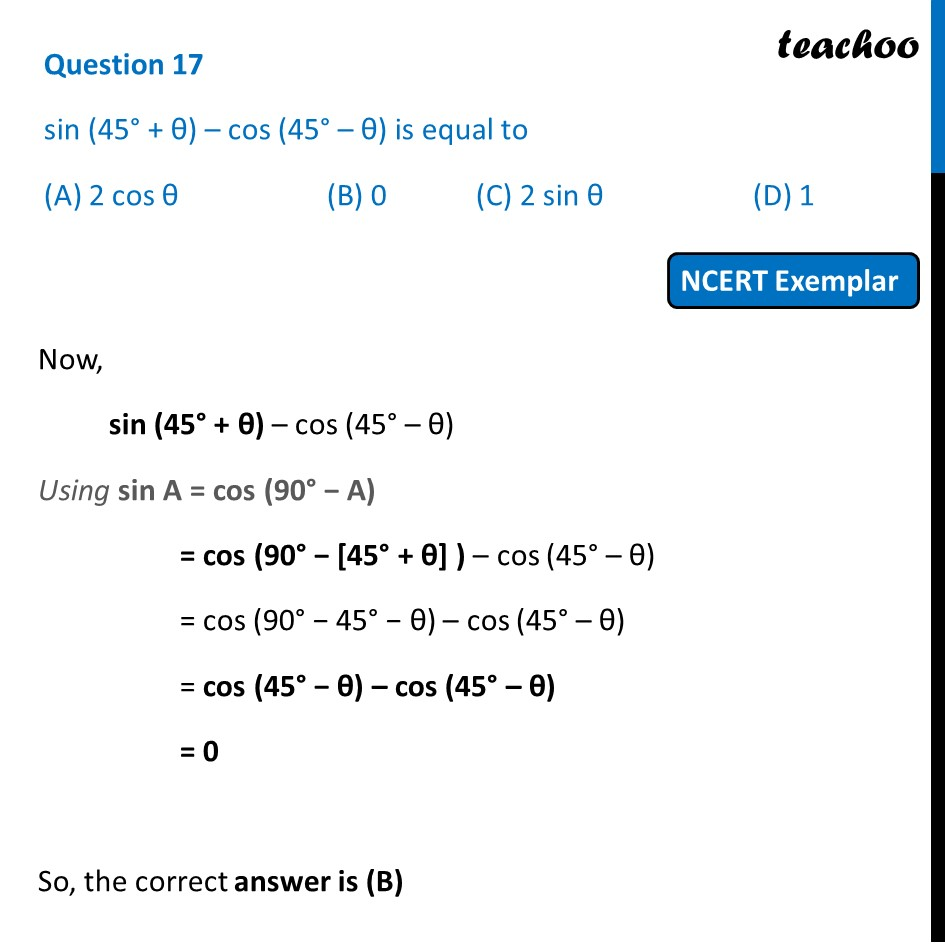(D) 11. Chapter 8 Class 10 Introduction to Trignometry (Term 1)
2. Serial order wise
3. NCERT Exemplar - MCQ

Transcript

Question 17 sin (45° + θ) – cos (45° – θ) is equal to (A) 2 cos θ (B) 0 (C) 2 sin θ (D) 1 Now, sin (45° + θ) – cos (45° – θ) Using sin A = cos (90° − A) = cos (90° − [45° + θ] ) – cos (45° – θ) = cos (90° − 45° − θ) – cos (45° – θ) = cos (45° − θ) – cos (45° – θ) = 0 So, the correct answer is (B)

NCERT Exemplar - MCQ

Chapter 8 Class 10 Introduction to Trignometry (Term 1)
Serial order wise